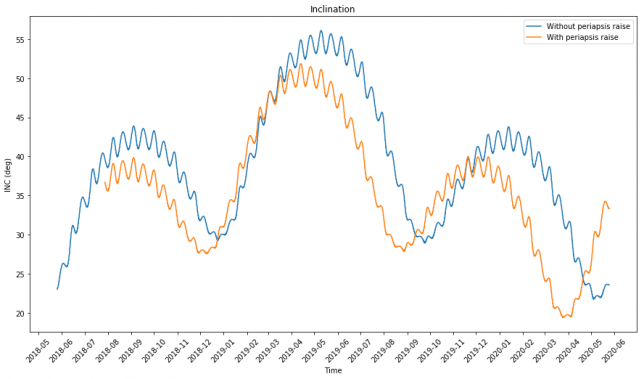# DSLWP-B long-term orbit evolution

In my last post I commented that one of the motivations for the periapsis raise manoeuvre of DSLWP-B on July 20 was to prevent the satellite from crashing into the Moon in a few months. When Wei Mingchuan BG2BHC told me this, I found it a bit surprising, since I had the impression that the periapsis had been raising naturally since the satellite was injected in lunar orbit on May 25. Thus, I decided to propagate the orbit for a period of 2 years using GMAT and study the long-term effects in the Keplerian elements.

In this study I’ll use two GMAT script files: orbit_evolution.script and orbit_evolution2.script. The first script uses the orbital state that I obtained from one month of S-band Doppler measurements made by Scott Tilley VE7TIL. Thus, this file represents the orbital state when the spacecraft was injected in lunar orbit. When we propagate this state we will see what would have happened if the periapsis raise hadn’t been performed. The orbital state for the second file is taken from the first line of the 20160727a tracking file in dslwp_dev. Therefore, this file represents the orbit after the periapsis raise, so that we can compare both orbits.

The force model used in GMAT considers lunar spherical harmonics up to degree and order 10, the point forces of the Sun, Earth and the remaining planets (except for Mercury and Pluto), solar radiation pressure (although I don’t have good estimates for the reflectivity coefficients) and relativistic effects. Tidal effects are not considered.

After running the scripts in GMAT and processing the results in this Jupyter notebook, we get the following plots. The first shows the periapsis radius, which is our main concern. For comparison, I have plotted the lunar radius in red.

We see that both Wei and I were right. For the time being, the periapsis radius has been increasing. However, it will start to decrease in September, and without the periapsis raise manoeuvre, DSLWP-B would impact the Moon on December. With the periapsis raise, the orbit misses the lunar surface by around 250km.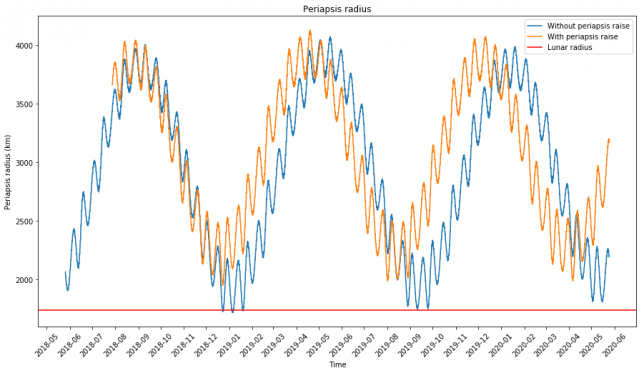The next figure shows the apoapsis radius.As we can see, the evolution of the periapsis and apoapsis is opposite. Both are afected by two periodic variations: one having a period of roughly half a lunar month, and the other having a period of around 8 months. I don’t know yet the precise cause of these periodic variations. Probably they are caused by some sort of resonance. Perhaps I’ll look at this again in the future.

The reason why the periapsis and apoapsis evolve in an opposite way is simple. The semi-major axis cannot change much, as it is closely related to the orbital energy (on the Keplerian two-body model it is equivalent to the orbital energy). Therefore, the changes in periapsis and apoapsis are mainly caused by a change in the eccentricity, which affects them with opposite sign. The eccentricity can be seen below.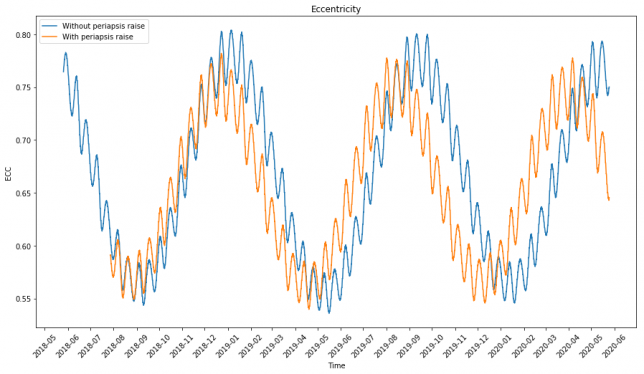The next figure shows the evolution of the right ascension of the ascending node. It also shows the same periodic variations as the other parameters, but the dominating factor is a secular variation that makes the ascending node complete a full turn roughly every two years. This precession of the orbital plane is a well understood phenomenon and it is mainly caused by the $$J_2$$ spherical harmonic (related to the oblateness of the Moon).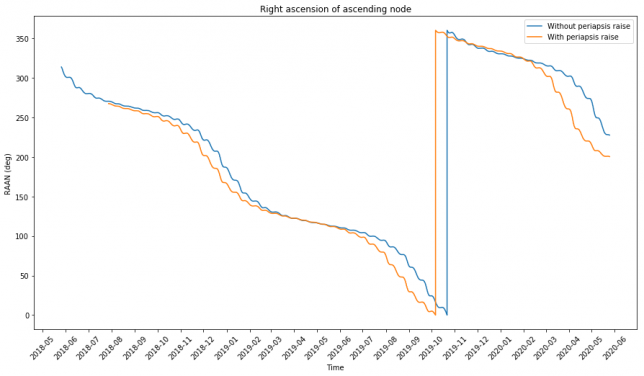The argument of periapsis shows the kind of same precession.Since the argument of periapsis is measured from the ascending node and the ascending node is preceeding, this is not a very good representation of the position of the periapsis. It is better to use the right ascension of the periapsis, shown below. It shows a smaller secular variation: a full turn would take around 7 years to complete. This precession of the periapsis is also well understood and related to $$J_2$$ (see here).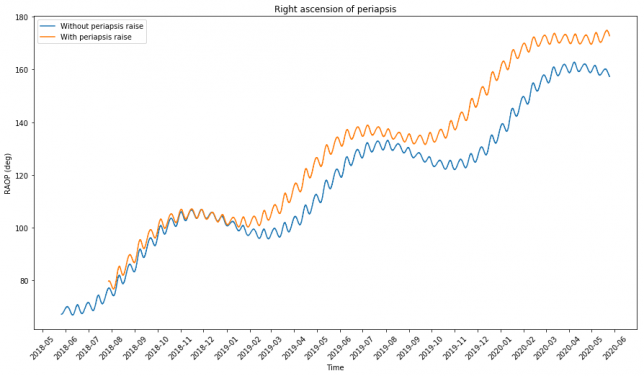Finally, we show the inclination. Its behaviour is quite interesting, and not completely explained as a superposition of the two periodic variations we have seen.